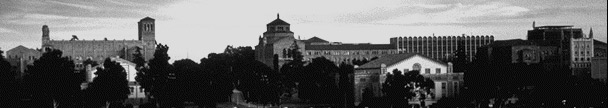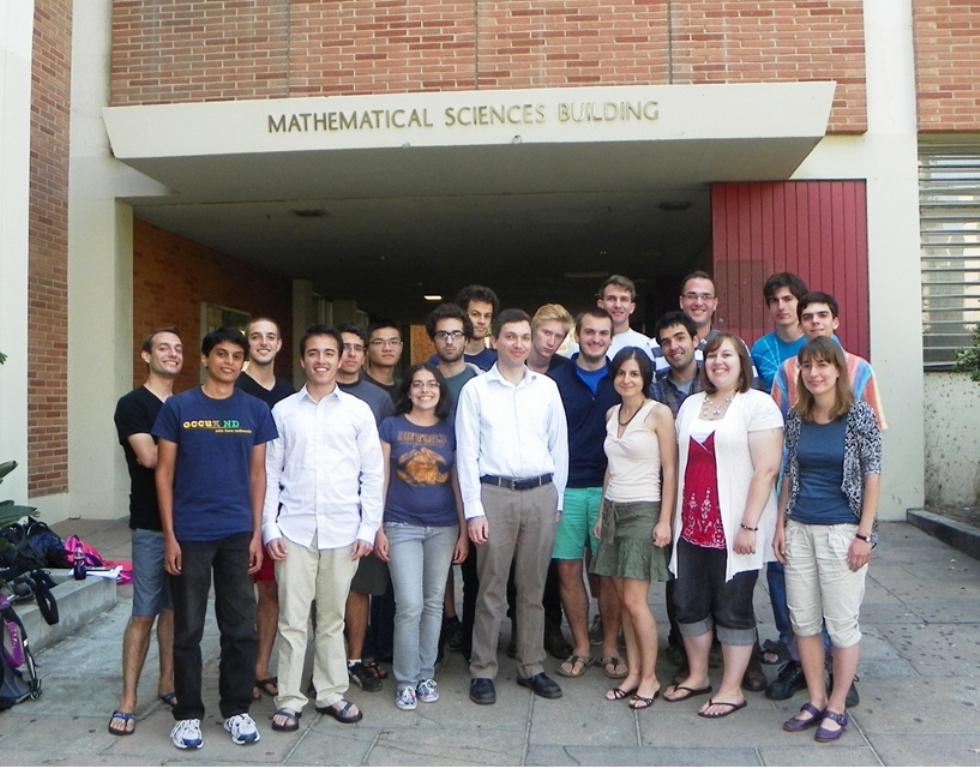UCLA Logic Center## (This is the 2012 page. Are you looking for the current Summer School?)

The UCLA Logic Center held a three-week summer school for undergraduates, from Sunday June 24, to Saturday July 14, 2012. (Summer school flyer, 8.5 x 11 inches, PDF format.)

The goal of the center's summer schools is to introduce future mathematicians to central results and techniques from mathematical logic. Courses are very intensive, designed to assume little if any prior experience with logic, yet reach highly advanced, graduate level material, within three weeks. They serve as good introduction to the kind of research work that students of mathematics can expect in graduate school.

Thanks to an NSF grant (DMS-1044604) and Logic Center support we were able to provide admitted students with a stipend of \$3000, travel allowance up to \$500, and dormitory housing at UCLA for no charge (double occupancy rooms, breakfast and dinner included).

Each course in the summer school met daily for two hours of lecture, and one hour of guided problem solving in small groups. Students each took two of the three courses offered. In addition to the six daily hours of course work (not to mention endless hours of extra work on challenging problem sets over evenings and weekends) there were lectures on topics of current research, social events, and planned outings in the area.### Topics in 2012:

First order logic and Gödel's incompleteness theorem
Instructor: Anush Tserunyan

At the beginning of the 20th century mathematics experienced a crisis due to the discovery of certain paradoxes, e.g. Russell's paradox, in previous attempts to formalize abstract notions of sets and functions. To put analysis on a firm foundation, similar to the axiomatic foundation for geometry, Hilbert proposed a program aimed at a direct consistency proof of analysis. This would involve a system of axioms that is consistent, meaning free of internal contradictions, and complete, meaning rich enough to prove all true statements. But the search for such a system was doomed to fail: Gödel proved in the early 1930s that any system of axioms that can be listed by some computable process, and subsumes Peano arithmetic, is either incomplete or inconsistent. This is the Gödel incompleteness theorem, and we will prove it in the second half of this course. In the first half, we will develop the framework of first order logic, culminating in a proof of the compactness theorem, one of the most useful tools of logic. In addition, we will discuss applications in various fields of mathematics such as combinatorics and algebra.

Forcing and independence in set theory
Instructor: Justin Palumbo

Set-theoretic forcing is a technique originally introduced by Paul Cohen to prove that the axiom of choice and the continuum hypothesis are independent of the classical axioms of mathematics. Since then it has led to an explosive growth in research, and has been used by set theorists to prove literally hundreds of independence results. In this course we will introduce students to the forcing technique by first studying some of the combinatorial consequences of Martin's Axiom, an axiom which when added to ZFC makes it possible to prove consistency results by means very similar to those used in forcing. We will then introduce forcing itself and show how it can be applied to settle the independence of the continuum hypothesis.

Non-standard analysis
Instructor: Isaac Goldbring

Infinitely small numbers and infinitely large numbers have been used as a heuristic tool in mathematics throughout the centuries: Archimedes used them to derive formulas for the areas and volumes of geometric figures, Newton and Leibniz used infinitesimals to develop the differential and integral calculus, and Euler employed infinitesimals to derive series expansions of logarithmic, exponential, and trigonometric functions. But the concept remained vague, and its use became unacceptable with the introduction of a rigorous foundation for analysis in the 19th century. In the early 1960s, the logician Abraham Robinson realized that techniques from mathematical logic, most notably the compactness theorem, could be used to give infinitesimal analysis a firm foundation. In this course, we will develop Robinson's rigorous non-standard methods, and use them to prove theorems from a wide spectrum of mathematical areas, including basic analysis, metric space topology, measure theory, commutative algebra, and combinatorics.